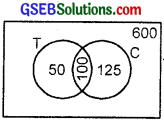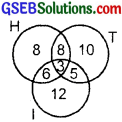# GSEB Solutions Class 11 Maths Chapter 1 Sets Miscellaneous Exercise

Gujarat Board GSEB Textbook Solutions Class 11 Maths Chapter 1 Sets Miscellaneous Textbook Questions and Answers.

## Gujarat Board Textbook Solutions Class 11 Maths Chapter 1 Sets Miscellaneous Exercise

Question 1.
Decide, among the following sets, which are the subsets of one and another: A = {x : x ∈ R and x satisfy x2 – 8x + 12 = 0}, B = {2, 4, 6}, C = {2, 4, 6, 8, …}, D = {6}.
Solution:
We have:
A = {2, 6}, B = {2, 4, 6}, C = {2, 4, 6, 8, …} and D = {6}.
Clearly, every element of A is in B and C.
∴ A ⊂ B and A ⊂ C.
Again, every element of B is in C.
∴ B ⊂ C.
Also, every element of D is in A, B and C.
∴ D ⊂ A, D ⊂ B and D ⊂ C.Question 2.
In each of the following, determine whether the statement is true or false. If it is true, prove it. If it is false, give an example?

1. If x ∈ A and A ∈ B, then x ∈ B.
2. If A ⊂ B and B ∈ C, then A ∈ C.
3. If A ⊂ B and B ⊂ C, then A ⊂ C.
4. If A ⊄ B and B ⊄ C, then A ⊄ C.
5. If x ∈ A and A ⊄ B, then x ∈ B.
6. If A ⊂ B and then x ∉ A.

Solution:
1. False. Let A = {1}, B = {{1}, 2}
Clearly, 1 ∈ A and A ∈ B but 1 ∉ B.
So, x ∈ A and A ∈ B need not imply that x ∈ B.

2. False. Let A = {1}, B = {1, 2} and C = {{1, 2}, 3}
Clearly, A ⊂ B and B ∈ C but A ∉ C
Thus, A ⊂ B and B ∈ C need not imply that A ∈ C.

3. True. Let x ∈ A. Then,
A ⊂ B ⇒ x ∈ B ⇒ x ∈ C.
Thus, x ∈ A ⇒ x ∈ C for all x ∈ A ⇒ A ⊂ C
Hence, A ⊂ B and B ⊂ C ⇒ A ⊂ C.

4. False. Let A = {1, 2}, B = {2, 3} and C = {1, 2, 5}
Then, A ⊄ B and B ⊄ C. But A ⊄ C.
Thus, A ⊄ B and B ⊄ C need not imply that A ⊄ C.

5. False. Let A = {1, 2} and B = {2, 3, 4, 5}
Clearly, 1 ∈ A and A ⊄ B but 1 ∉ B.
Thus, x ∈ A and A ⊄ B need not imply that x ∈ B.

6. True. Let A ⊂ B. Then, clearly x ∈A ⇒ x ∈ B ⇔ x ∉ B
⇒ x ∉ A.Question 3.
Let A, B and C be the sets such that A ∪ B = A ∪ C and A ∩ B = A ∩ C. Show that B = C.
Solution:
We have A ∪ B = A ∪ C.
⇒ (A ∪ B) ∩ C = (A ∪ C) ∩ C
⇒ (A ∩ C) ∪ (B ∩ C) = C [∵ (A ∪ C) ∩ C = C]
⇒ (A ∪ B) ∩ (B ∩ C) = C …………….. (1) [∵ A ∩ C = A ∩ B]
Again, A ∪ B = A ∪ C
⇒ (A ∪ B) ∩ B = (A ∪ C) ∩ B
⇒ B = (A ∩ B) ∪ (C ∩ B) [∵ (A ∪ B) ∩ B = B]
⇒ B = (A ∩ B) ∪ (B ∩ C) …………….. (2)
From (1) and (2), we get
B = C.

Question 4.
Show that the following four conditions are equivalent.
(i) A ⊂ B
(ii) A – B = ϕ
(iii) A ∪ B = B
(iv) A ∩ B = A.
Solution:
(i) ⇔ (ii)
A ⊂ B ⇔ All elements of A are inB ⇔ A – B = ϕ.
(ii) ⇔ (iii)
A – B = ϕ ⇔ All the elements of A are in B ⇔ A ∪ B = B.
(iii) ⇔ (v)
A ∪ B = B ⇔ All the elements of A are in B.
⇔ All the elements of A are common in A and B ⇔ A ∩ B = A.
Thus, all the four given conditions are equivalent.Question 5.
Show that if A ⊂ B, then C – B ⊂ C – A.
Solution:
x ∈ C – B ⇔ x ∈ C but x ∉ B
⇒ x ∈ C but x ∉ A
⇒ x ∈ C – A
∴ C – B ⊂ C – A

Question 6.
Assume that P(A) = P(B). Prove that A = B?
Solution:
Let x be an arbitrary element of A. Then, there exists a subset, say X, of set A such that x ∈ X.
Now, X ⊂ A ⇒ X ∈ P(A)
⇒ X ∈ P(B) [∵ P(A) = P(B)]
⇒ X ⊂ B
⇒ x ∈ B [∵ x ∈ X and X ⊂ B ⇒ x ∈ B}
Thus, x ∈ A ⇒ x ∈ B
∴ A ⊂ B. ……………………. (1)
Now, let y be an arbitrary element of B. Then, there exists a subset, say Y, of set B such that y ∈ Y.
Now, Y ⊂ B ⇒ Y ∈ P(B).
⇒ Y ∈ P(A)
⇒ Y ⊂ A ⇒ Y ∈ A.
Thus, y ∈B ⇒ y ∈ A
∴ B ⊂ A.
From (1) and (2), we get
A = B.Question 7.
Is it true that, for any sets A and B, P(A) ∪ P(B) = P(A ∪ B)? Justify your answer?
Solution:
No.
Let A = {a}, B = {b} and A ∪ B = {a, b}.
∴ P(A) = {ϕ, {a}},P(B) = {ϕ, {b}}
and P(A ∪ B) = {ϕ, {a}, {b}, {a, b}} …………………… (1)
and P(A) ∪ P(B) = {ϕ, {a}, {b}} ………………… (2)
From (1) and (2), we have:
P(A ∪ B) ≠ P(A) ∪ P(B).

Question 8.
Show that for any sets A and B, A = (A ∩ B) ∪ (A – B) and A ∪ (B – A) = A ∪ B?
Solution:
(A ∩ B) ∪ (A – B) = (A ∩ B) ∪ (A ∩ B’)[∵A – B = A ∩ B’]
= A ∩ (B ∪ B’) [Distributive law]
= A ∩ X, where X is universal set
= A.
A ∪ (B – A) – A ∪ (B ∩ A’)
= (A ∪ B) ∩ (A ∪ A’) [Distributive law]
= (A ∪ B) ∩ X,
where X = A ∪ A’ is universal set
= A ∪ B [∵ A ∪ B ⊂ X]Question 9.
Using properties of sets, show that

1. A ∪ (A ∩ B) = A
2. A ∩ (A ∪ B) = A

Solution:
1. A ∪ (A ∩ B) = (A ∪ A) ∩ (A ∪ B) [Distributive law]
= A ∩ (A ∪ B) [∵ A ∪ A = A]
= A [∵ A ⊆ A ∪B]

2. A ∩ (A ∪ B) = (A ∩ A) ∪ (A ∩ B)
= A ∪ (A ∩ B)
= A [∵ A ∩ B ⊂ A]

Question 10.
Show that A ∩ B = A ∩ C need not imply B = C?
Solution:
With the help of an example, we may try to establish it.
Let A = {1, 2}, B = {1, 3} and C = (1, 4).
Now, A ∩ B = {1, 2} ∩{1, 3} = {1}
and A ∩ C = (1, 2) ∩ {1, 4} = {1}.
∴ A ∩ B = A ∩ C
still B ≠ C.

Question 11.
Let A and B be sets. If A ∩ X = B ∩ X = ϕ and A ∪ X = B ∪ X for some set X, prove that A = B?
Solution:
We have:
A ∪ X = B ∪ X for some set X.
⇒ A ∩ (A ∪ X) = A ∩ (B ∪ X).
⇒ A = (A ∩ B) ∪ (A ∩ X) [∵ A ∩ (A ∪ X) = A]
⇒ A = (A ∩ B) ∪ ϕ [∵ A ∩ X = ϕ (given)] …………………. (1)
⇒ A = A ∩ B ⇒ A ⊂ B
Again, A ∪ X = B ∪ X.
⇒ B ∩ (A ∪ X) = B ∩ (B ∪ X) [∵ B ∩ (B ∪ X) = B]
⇒ (B ∩ A) ∪ ϕ = B ⇒ B ∩ A = B
⇒ A ∩ B = B ⇒ B ⊂ A ……………………. (2)
From (1) and (2), we get
A = B.Question 12.
Find sets A, B and C such that A ∩ B, A ∩ C and B ∩ C are non-empty sets and A ∩ B ∩ C = ϕ.
Solution:
Let A = {1, 2}, B = {2, 3} and C = {1, 3).
Clearly, A ∩ B = {2), B ∩ C = (3) and A ∩ C = {1}.
i.e., A ∩ B, A ∩ C and B ∩ C are non-empty sets.
∴ (A ∩ B) ∩ C = (2) ∩ (1, 3) A ∩ B ∩ C = ϕ

Question 13.
In a survey of 600 students in a school, 150 students were found to be drinking Tea and 225 drinking Coffee, 100 were drinking both Tea and Coffee. Find how many students were drinking neither Tea nor Coffee?
Solution:
Here, n(T) = 150, n(C) = 225, n(T ∩ C) = 100.
Also, we know that
n(T ∪C) = n(T) + n(C) – n(T ∩C)
= 150 + 225 – 100
= 275.
Total no. of students = 600.
∴ No. of students who neither take tea nor coffee
= 600 – n(T ∪C)
= 600 – 275
= 325.Question 14.
In a group of students, 100 students know Hindi, 50 know English and 25 know both. Each of the students knows either Hindi or English. How many students are there in the group?
Solution:
Here, n(H) = 100, n(E) = 50
and n(H ∩ E) = 25.We know that
n(H ∪E) = n(H) + n(E) – n(H ∩E)
= 100 + 50 – 25
= 125.

Question 15.
In a survey of 60 people, it was found that 25 people read Newspaper H, 26 read Newspaper I, 26 read Newspaper I, 9 read both H and I, 11 read both H and T, 8 read both T and I, 3 read all three newspapers. Find?

1. The number of people who read at least one of the newspapers.
2. The number of people who read exactly one newspaper.

Solution:
Clearly,
1. No. of people who read at least one newspaper
= (8 + 8 + 10 + 6 + 3 + 5 + 12)
= 52.

2. No. of people who read exactly
one newspaper = 52 – (8 + 3 + 6 + 5)
= 52 – 22
= 30.Question 16.
In a survey, it was found that 21 people liked product A, 26 liked product B and 29 liked product C. If 14 people liked A and B, 12 people liked C and A, 14 people liked product B and C and 8 liked all the three products, find how many liked product C only?
Solution:
n(A ∩ B) = 14, n(A ∩ C) = 12,
n(B ∩ C) = 14,
n(A ∩ B ∩ C) = 8.
∴ n(C only) = 29 – 4 – 8 – 6
= 29 – 18
= 11.